# WVPlots examples

#### 2023-08-19

Some example data science plots in R using ggplot2. See https://github.com/WinVector/WVPlots for code/details.

set.seed(34903490)
x = rnorm(50)
y = 0.5*x^2 + 2*x + rnorm(length(x))
frm = data.frame(
x = x,
y = y,
yC = y>=as.numeric(quantile(y,probs=0.8)),
stringsAsFactors = FALSE)
frm$absY <- abs(frm$y)
frm$posY = frm$y > 0

## Scatterplots

Scatterplot with smoothing line through points.

WVPlots::ScatterHist(frm, "x", "y", title="Example Fit")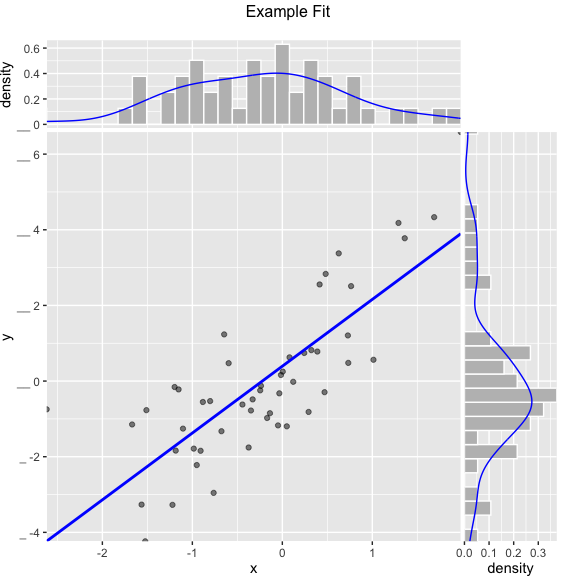Scatterplot with best linear fit through points. Also report the R-squared and significance of the linear fit.

WVPlots::ScatterHist(frm, "x", "y", smoothmethod="lm",
title="Example Linear Fit", estimate_sig = TRUE)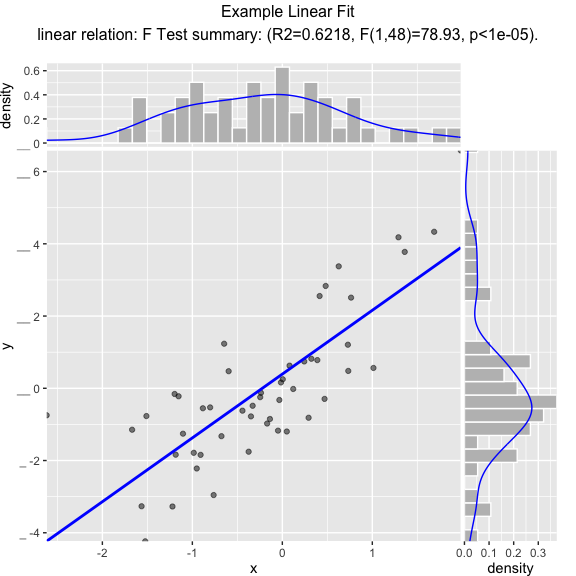Scatterplot compared to the line x = y. Also report the coefficient of determination between x and y (where y is “true outcome” and x is “predicted outcome”).

WVPlots::ScatterHist(frm, "x", "y", smoothmethod="identity",
title="Example Relation Plot", estimate_sig = TRUE)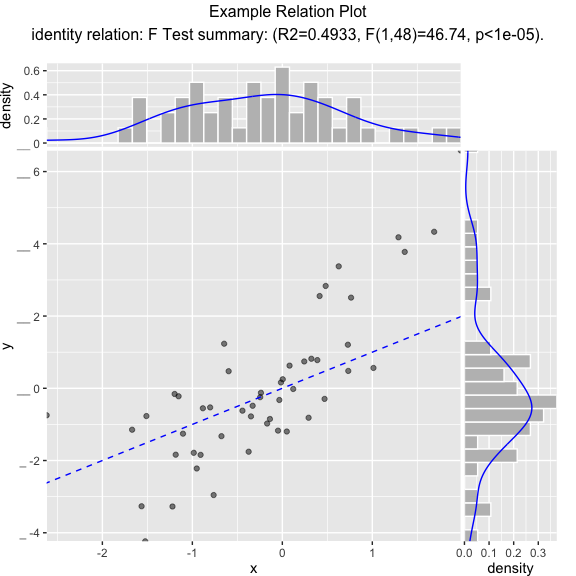Scatterplot of (x, y) color-coded by category/group, with marginal distributions of x and y conditioned on group.

set.seed(34903490)
fmScatterHistC = data.frame(
x=rnorm(50),
y=rnorm(50),
stringsAsFactors = FALSE)
fmScatterHistC$cat <- fmScatterHistC$x+fmScatterHistC$y>0 WVPlots::ScatterHistC(fmScatterHistC, "x", "y", "cat", title="Example Conditional Distribution")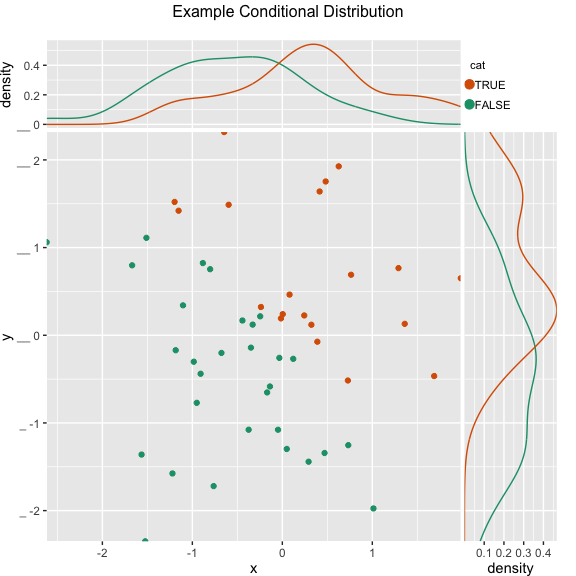Scatterplot of (x, y) color-coded by discretized z. The continuous variable z is binned into three groups, and then plotted as by ScatterHistC set.seed(34903490) frmScatterHistN = data.frame( x=rnorm(50), y=rnorm(50), stringsAsFactors = FALSE) frmScatterHistN$z <- frmScatterHistN$x+frmScatterHistN$y
WVPlots::ScatterHistN(frmScatterHistN, "x", "y", "z", title="Example Joint Distribution")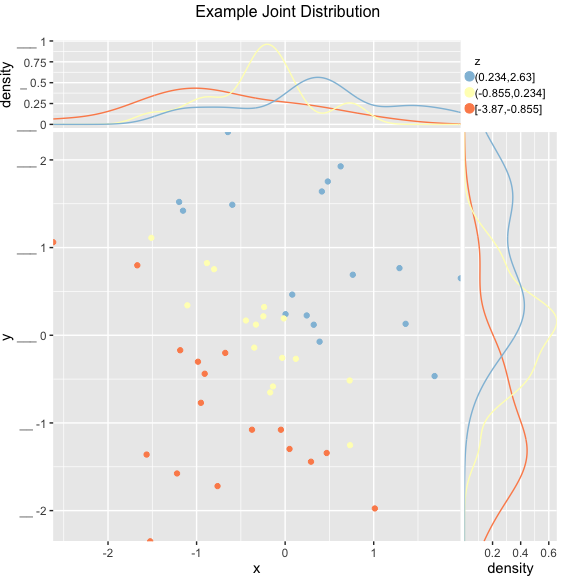Plot the relationship y as a function of x with a smoothing curve that estimates $$E[y | x]$$. If y is a 0/1 variable as below (binary classification, where 1 is the target class), then the smoothing curve estimates $$P(y | x)$$. Since $$y \in \{0,1\}$$ with $$y$$ intended to be monotone in $$x$$ is the most common use of this graph, BinaryYScatterPlot uses a glm smoother by default (use_glm=TRUE, this is essentially Platt scaling), as the best estimate of $$P(y | x)$$.

WVPlots::BinaryYScatterPlot(frm, "x", "posY", use_glm=FALSE,
title="Example 'Probability of Y' Plot (ggplot2 smoothing)")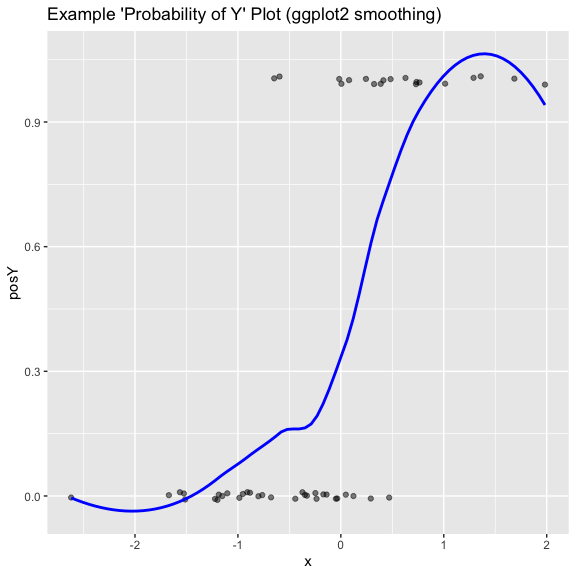WVPlots::BinaryYScatterPlot(frm, "x", "posY", use_glm=TRUE,
title="Example 'Probability of Y' Plot (GLM smoothing)")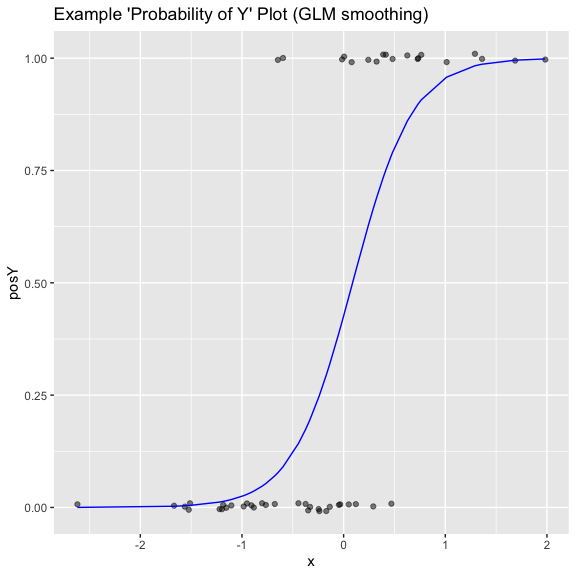## Hexbin Plot

if(requireNamespace("hexbin", quietly = TRUE)) {
set.seed(5353636)

df = rbind(data.frame(x=rnorm(1000, mean = 1),
y=rnorm(1000, mean = 1, sd = 0.5 ),
stringsAsFactors = FALSE),
data.frame(x = rnorm(1000, mean = -1, sd = 0.5),
y = rnorm(1000, mean = -1, sd = 0.5),
stringsAsFactors = FALSE),
stringsAsFactors = FALSE)

print(WVPlots::HexBinPlot(df, "x", "y", "Two gaussians"))
}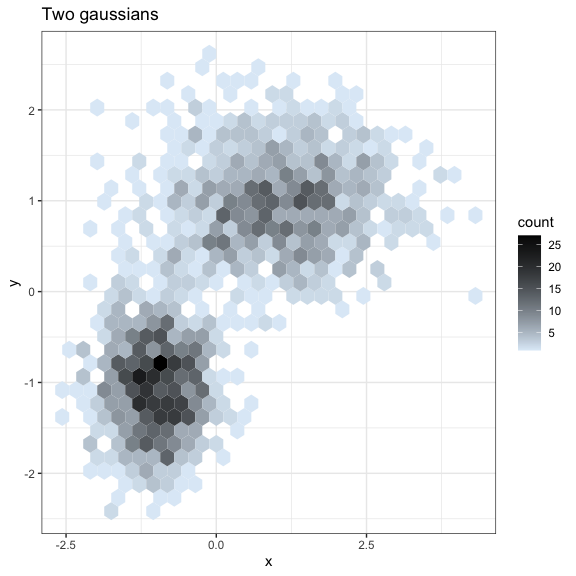## Gain Curves

set.seed(34903490)
y = abs(rnorm(20)) + 0.1
x = abs(y + 0.5*rnorm(20))

frm = data.frame(
model=x,
value=y,
stringsAsFactors = FALSE)

frm$costs=1 frm$costs=5
frm$rate = with(frm, value/costs) frm$isValuable = (frm$value >= as.numeric(quantile(frm$value, probs=0.8)))

Basic curve: each item “costs” the same. The wizard sorts by true value, the x axis sorts by the model, and plots the fraction of the total population.

WVPlots::GainCurvePlot(frm, "model", "value", title="Example Continuous Gain Curve")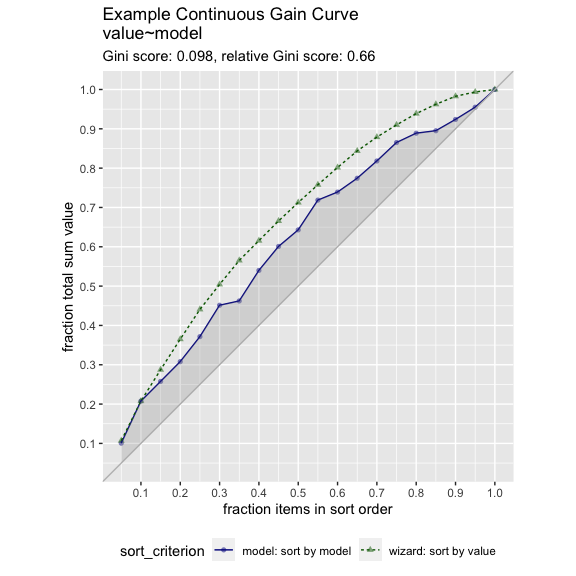We can annotate a point of the model at a specific x value

gainx = 0.10  # get the top 10% most valuable points as sorted by the model

# make a function to calculate the label for the annotated point
labelfun = function(gx, gy) {
pctx = gx*100
pcty = gy*100

paste("The top ", pctx, "% most valuable points by the model\n",
"are ", pcty, "% of total actual value", sep='')
}

WVPlots::GainCurvePlotWithNotation(frm, "model", "value",
title="Example Gain Curve with annotation",
gainx=gainx,labelfun=labelfun)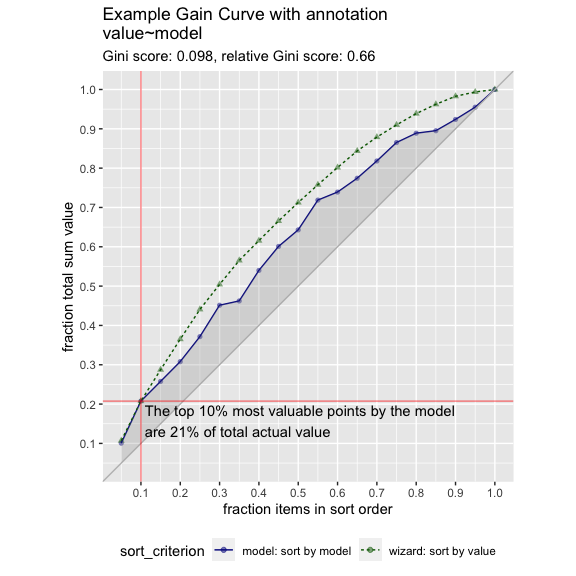When the x values have different costs, take that into account in the gain curve. The wizard now sorts by value/cost, and the x axis is sorted by the model, but plots the fraction of total cost, rather than total count.

WVPlots::GainCurvePlotC(frm, "model", "costs", "value", title="Example Continuous Gain CurveC")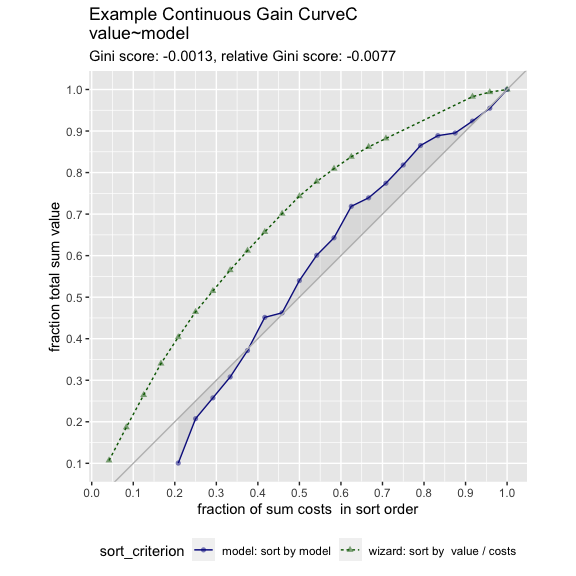## ROC Plots

set.seed(34903490)

# data with two different regimes of behavior
frm <- rbind(
data.frame(
model = rnorm(1000),
isValuable = sample(c(TRUE, FALSE), prob = c(0.02, 0.98), size = 1000, replace = TRUE)),
data.frame(
model = rnorm(200) + 5,
isValuable = sample(c(TRUE, FALSE), size = 200, replace = TRUE))
)

WVPlots::ROCPlot(frm, "model", "isValuable", TRUE, title="Example ROC plot")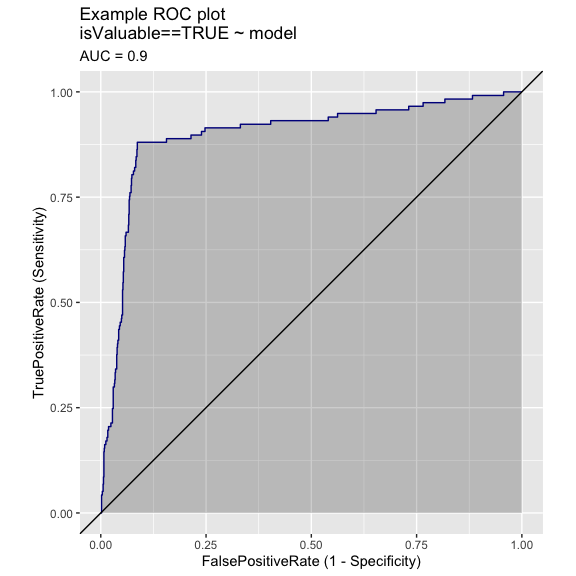Plotting the ROC of two models on the same data, where predictions and true outcome all in the same data frame.

set.seed(34903490)

x1 = rnorm(50)
x2 = rnorm(length(x1))
y = 0.2*x2^2 + 0.5*x2 + x1 + rnorm(length(x1))
frmP = data.frame(
x1=x1,
x2=x2,
yC = y>=as.numeric(quantile(y,probs=0.8)),
stringsAsFactors = FALSE)
# WVPlots::ROCPlot(frmP, "x1", "yC", TRUE, title="Example ROC plot")
# WVPlots::ROCPlot(frmP, "x2", "yC", TRUE, title="Example ROC plot")
WVPlots::ROCPlotPair(frmP, "x1", "x2", "yC", TRUE, title="Example ROC pair plot")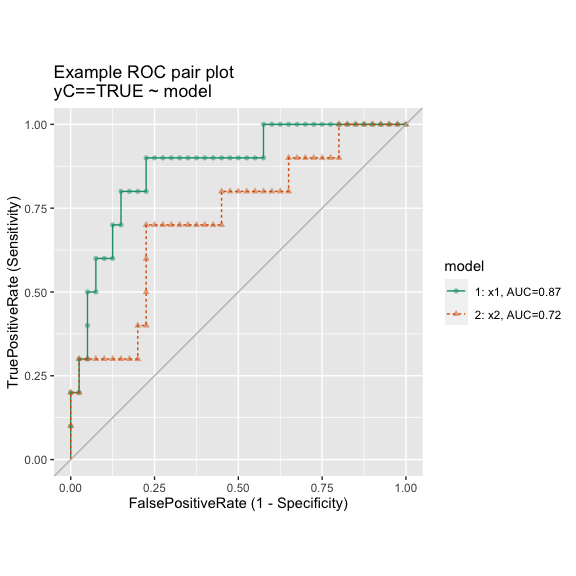Plotting the results from two data sets, for example the results of a model on training and test sets, where predictions/outcome for the two data sets are in different data frames.

set.seed(2342458)

make_data <- function(nrows) {
d <- data.frame(x = rnorm(nrows))
d['y'] = sin(d['x']) + 0.25*rnorm(n = nrows)
d['x2'] = rnorm(n = nrows)
d['yc'] = d[['y']]>0.5
return(d)
}

training <- make_data(500)
test <- make_data(200)

model <- glm(yc ~ x + x2, data=training, family=binomial)

training$pred <- predict(model, newdata=training, type="response") test$pred <- predict(model, newdata=test, type="response")

WVPlots::ROCPlotPair2(nm1 = "Training", # model 1
frame1 = training,
xvar1 = "pred",  truthVar1 = "yc", truthTarget1 = TRUE,
nm2 ="Test", # model 2
frame2 = test,
xvar2 = "pred", truthVar2 = "yc", truthTarget2 = TRUE,
title = "Model performance, training vs test",
estimate_sig = FALSE)